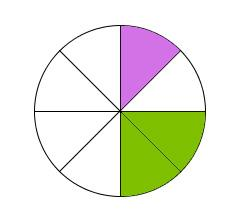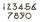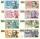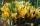# Unknown number

Find the unknown number equal to a quarter of a fifth of a number, which is by 152 more than an unknown number.

x =  8

### Step-by-step explanation:

x=1/4•1/5•(152+x)

19x = 152

x = 8

Our simple equation calculator calculates it.Did you find an error or inaccuracy? Feel free to write us. Thank you!Tips to related online calculators
Need help to calculate sum, simplify or multiply fractions? Try our fraction calculator.
Do you have a linear equation or system of equations and looking for its solution? Or do you have a quadratic equation?

## Related math problems and questions:

• Unknown number 6Determine the unknown number, which is by 1.5 greater than its fourth.
• Unknown xIf we add to unknown number 21, then divide by 6 and then subtract 51, we get back an unknown number. What is this unknown number?
• Unknown number 6Determine x if 1/6 of x is equal to 2/5 of the number 24.
• NumberWhat number do I think? The third is 6, and a half is 2 more than its quarter.
• Find unknown numberWhat is the number between 50 and 55 that is divisible by 2,3,6,9?
• UN 1If we add to an unknown number his quarter, we get 210. Identify unknown number.
• By sixFrom the digits 1,2,3,4 we create the long integer number 123412341234. .. .. , which will have 962 digits. Is this number divisible by 6?
• Unknown numberFind an unknown number of which 1/5 is 40 greater than one-tenth of that number.
• Unknown numberI think the number. I'll reduce it to its one-third. The result is then increased by one-third, and I get the number 12.
• One-thirdA one-third of unknown number is equal to five times as great as the difference of the same unknown number and number 28. Determine the unknown number.
• Unknown numberSamuel wrote unknown number. Then he had add 200000 to the number and the result multiply by three. When it calculated he was surprised, because the result would have received anyway, if write digit to the end of original number. Find unknown number.
• Unknown number 5I think of an unknown number. If we enlarge it five times, then subtract 3, and the result decreases by 75%, we get one greater than the number. What number am I thinking of?
• Cash backAfter a trip 24 students have left more than 650 but less than 690 CZK (Kc). How much each student receives return when the amount is divisible by the number of pupils?
• CrocusesThe garden grows daffodils, crocuses, and roses. 1400 daffodils, crocuses are 462 more, and roses are 156 more than crocuses. How many are all the flowers in the garden?
• I think numberI think number.When I add 841 to it and subtract 157, I get a number that is 22 greater than 996. What number I thinking?
• OrchardOne-eighth of the trees in the fruit plant in winter froze, and one-twelfth of damaged disease and pests. Healthy trees remained 152. Is it enough to supply 35 trees to restore the original number of trees in the orchard?
• Find unknown 2Find unknown denominator: 2/3 -5/? = 1/4